# Input and Output in Python

## Input and Output in Python with Examples

In this article, I am going to discuss Input and Output in Python with Examples. Please read our previous article where we discussed Operators in Pythonwith examples. At the end of this article, you will understand the following pointers in detail.

1. Why should we learn about input and output in python?
2. Input and output
3. Convert from String to type into other types
4. eval() function
5. Command-line arguments
6. IndexError
7. Len() function
8. Multiple Programs to understand the above concepts
##### Why the Input and Output chapter in Python?

In the previous chapters, all the coding examples were having values hardcoded within the code itself. Let’s have a look at the example below which checks if the person is in their teenage or not.

###### Example: Hardcoded values to a variable
```age = 16
if age >= 13 and age <= 19:
print("Teenage")```

Output: Teenage

In the above program, we have hard-coded the age value as 16. If we want to check for other ages then we need to open the file, change the value of age and then execute it again. This is not a good practice.

In real-time passing the values to the variables during runtime or dynamically is a good practice. So, it’s very necessary and important for us to know about all the functions or methods available in python to take the input during runtime.

##### INPUT and OUTPUT in Python

A predefined function input() is available in python to take input from the keyboard during runtime. This function takes a value from the keyboard and returns it as a string type. Based on the requirement we can convert from string to other types.

###### Example: input() method in Python
```name = input("Enter the name: ")
print("You entered name as: ", name)```
###### Output: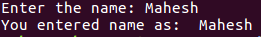###### Example: Checking return type of input() method in python
```name = input("Enter the name: ")
age = input("Enter the age: ")
print("You entered name as: ", name)
print("You entered age as:", age)
print(type(age))```
###### Output:In the above example, the type of age is a string but not an integer. If we want to use it as an integer then we need to do type conversion on it and then use it. Let’s understand that through our teenager program.

##### Example: Checking return type of input() method in python
```age = input("Enter the age: ")
if int(age) >= 13 and int(age) <= 19:
print("Teenage")```
###### Output: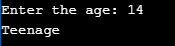##### Convert to other data types from a string in Python

We can convert the string to other data types using some inbuilt functions. We shall discuss all the type conversion types in the later chapters. As far as this chapter is concerned, it’s good to know the below conversion functions.

1. string to int – int() function
2. string to float – float() function
##### Example: Converting string type to int() in Python
```age = input("Enter your age: ")
print("age type is: ", type(age))

x = int(age)
print("After converting from string to int your age is: ", x)
print("now age type is: ", type(x))```
###### Output: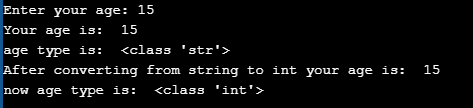##### Example: Converting string type to float() in Python
```salary = input("Enter your salary: ")
print("salary type is: ", type(salary))

x = float(salary)
print("After converting from string to float your salary is: ", x)
print("now salary type is: ", type(x))```
###### Output:##### Example: Taking int value at run time in Python
```age = int(input("Enter your age: "))
print("age type is: ",type(age))```
###### Output:##### Example: Taking int value and print their sum in Python
```x = int(input("Enter first number: "))
y = int(input("Enter second number: "))
print("Sum of two values: ", x+y)```
###### Output: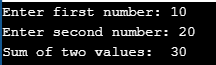##### eval() function in python

This is an in-built function available in python, which takes the strings as an input. The strings which we pass to it should, generally, be expressions. The eval() function takes the expression in the form of a string and evaluates it and returns the result.

###### Examples,
• eval(’10 + 10′) → 20
• eval(’10 * 10′) → 100
• eval(’10 and 10′) → 10
• eval(’10 or 10′) → 10
• eval(‘0 and 10’) → 0
• eval(’10 or 0′) → 10
• eval(’10 / 10′) → 1.0
• eval(’10 // 10′) → 1
• eval(’10 >= 10′) → True
##### Example: eval() function example in python
```sum = eval(input("Enter expression: "))
print(sum)```
###### Output: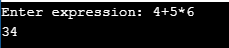###### Example: eval() function example in Python
```output = eval(input("Enter expression: "))
print(output)```
###### Output: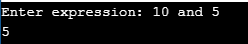##### Command Line Arguments in Python:

The command which we are using for running the python file for all the examples in this tutorial is “python filename.py”. This command we are executing thorough is command prompt/terminal. The script is invoked with this command and the execution starts. While invoking the script we can pass the arguments to it, which are called command-line arguments. Arguments are nothing but parameters or values to the variables.

The command with the arguments will look like: python filename.py 10 20

Our command is ‘python’ and the ‘filename.py’ ‘10’ ‘20’ are arguments to it. In order to use these arguments in our script/code, we should do the following import

from sys import argv

After including the above import statement in our file, the arguments which we pass from the command are available in an attribute called ‘argv’. argv will be a list of elements that can be accessed using indexes.

1. argv will always be the filename.py
2. argv will be 10 in this case
3. argv will be 20 in this case
##### Example: Command line arguments in python

demo11.py

```from sys import argv
print(argv)
print(argv)
print(argv)```

Command: python demo11.py 30 40
Output: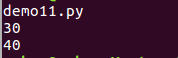##### IndexError in Python:

If we are passing 2 elements as arguments to the script (excluding the default argument filename), then the script has three arguments passed to it. But in the script, if we are trying to access the 10th argument with argv, then we get an IndexError. We can understand it from demo12.py

###### Example: Command line arguments

demo12.py

```from sys import argv
print(argv)```

Command: python demo12.py 30 40
Output: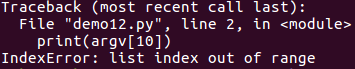Note: Command-line arguments are taken as strings elements, which means argv, argv, etc.. returns string type. (‘argv’ is a list as mentioned above and each individual element in it will be a string). Based on the requirement we can convert from string type to another type.

##### Example: Command line arguments

demo13.py

```from sys import argv
first_name = argv
last_name=argv
print("First name is: ", first_name)
print("Last name is: ", last_name)
print("Type of first name is", type(first_name))
print("Type of last name is", type(last_name))```

Command: python demo13.py Ram Pothineni
Output:##### Example: Command line arguments

demo14.py

```from sys import argv
item1_cost = argv
item2_cost =argv
print("First item cost is: ", item1_cost)
print("Second item cost is: ", item2_cost)
print("Type of item1_cost is : ", type(item1_cost))
print("Type of item2_cost is : ", type(item2_cost))
total_items_cost= item1_cost + item2_cost
print("Total cost is: ", total_items_cost)```

Command: python demo14.py 111 223
Output: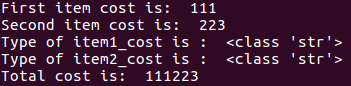##### Example: Command line arguments

demo15.py

```from sys import argv
item1_cost = argv
item2_cost =argv
x=int(item1_cost)
y=int(item2_cost)
print("First item cost is: ", x)
print("Second item cost is: ", y)
print("Type of item1_cost is : ", type(x))
print("Type of item2_cost is : ", type(y))
total_items_cost= x + y
print("Total cost is: ", total_items_cost)```

Command: python demo15.py 111 223
Output: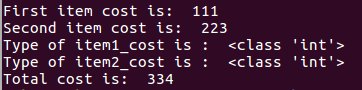In the demo14.py example, the requirement is to take the cost of the items and return the sum of it. For adding the two values we have used the ‘+’ operator which adds the two operands if they are integers and combines the two operands if they are strings. Since argv and agrv are taken as strings, the output is the addition of two strings ‘111’ and ‘223’ i.e ‘111223’.

In the demo15.py, we see that argv and agrv are converted to integer types and then the ‘+’ operator is applied to them. Hence the output in demo15.py is 334 i.e 111+223

##### len() function in python:

len() is an in-built function available in python, which can be applied on certain data types like lists, tuples, strings, etc in python to know how many elements are present in it. Since ‘argv’ is a list we can apply this len() function to it to know how many arguments have been passed.

##### Example: Command line arguments with len function in python

demo16.py

```from sys import argv
print("The length of values :", len(argv))```

Command: python demo16.py 10 20 30
Output: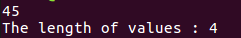Note: By default, space is the separator between two arguments while passing. If we want space in the argument itself, then we need to pass that argument enclosed in double-quoted( not in single quotes)

##### Example: Command line arguments

demo17.py

```from sys import argv
print(argv)```

Command: python demo17.py hello good morning
Output: hello

##### Example: Command line arguments

demo18.py

```from sys import argv
print(argv)```

Command: python demo18.py “hello good morning”
Output: hello good morning

### 2 thoughts on “Input and Output in Python ”

1.Raphael Ogunsanmi

This tutorial is helpful. I am new with programming and I am doing it with my Android phone.

I want to thank you for putting this together free for everyone.

Please I have a question and I need help . On
Command Line Arguments in Python:,
from sys import argv
first_name = argv
last_name=argv
print(“First name is: “, first_name)
print(“Last name is: “, last_name)
print(“Type of first name is”, type(first_name))
print(“Type of last name is”, type(last_name))
I am not getting a head way on this using my phone, please what do I do?

This is the results I have been getting.
Traceback (most recent call last):
File “/data/user/0/ru.iiec.pydroid3/files/accomp_files/iiec_run/iiec_run.py”, line 31, in
start(fakepyfile,mainpyfile)
File “/data/user/0/ru.iiec.pydroid3/files/accomp_files/iiec_run/iiec_run.py”, line 30, in start
File “”, line 1, in
ImportError: cannot import name ‘expressionpy’ from ‘sys’ (unknown location)

[Program finished]

Thanks in anticipation.

2.Raphael Ogunsanmi

This program is not working on my phone please what do I need to do?

from sys import argv
first_name = argv
last_name=argv
print(“First name is: “, first_name)
print(“Last name is: “, last_name)
print(“Type of first name is”, type(first_name))
print(“Type of last name is”, type(last_name))

This is the results I obtained using my phone.

Traceback (most recent call last):
File “/data/user/0/ru.iiec.pydroid3/files/accomp_files/iiec_run/iiec_run.py”, line 31, in
start(fakepyfile,mainpyfile)
File “/data/user/0/ru.iiec.pydroid3/files/accomp_files/iiec_run/iiec_run.py”, line 30, in start